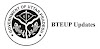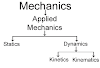# Up Polytechnic Applied physics-I download [pdf] | applied physics -I polytechnic pdf

## Up Polytechnic Applied physics-I download | applied physics -I polytechnic pdf

Applied Physics PDF includes the study of a diversified topics related to the world around us. It aims to give an understanding of this world both by observation and by prediction of the way in which objects behave. Concrete knowledge of physical laws, analysis and applications in various fields of Engineering Physics PDF and technology are given prominence in this course content.

Up Polytechnic Applied physics-I download [pdf] | applied physics -I polytechnic pdf
1. Define wave motion its types (Transverse and Longitudinal), Periodic and Simple Harmonic Motion, solve simple problems.
2. Define the terms: frequency, amplitude, wavelength, velocity of a wave.
3. Explain various Engineering Physics PDF, Medical and Industrial applications of Ultrasonic’s.
4. Apply acoustics principles to various types of buildings to get best sound effect
5. Explain diffraction, interference, polarization.
6. Define capacitance and its unit. They will be able to explain the function of capacitors in simple circuits, solve simple problems using C=Q/V
7. Explain the role of free electrons in insulators, conductors and semiconductors, qualitatively the terms: potential, potential difference, electromotive force.
8. Explain the concept of electric current, resistance and its measurement.
9. List the effects of an electric current and their common applications, State and apply Ohm’s law, calculate the equivalent resistance of a variety of resistor combinations, determine the energy consumed by an appliance, differentiate between AC electricity and DC electricity

Previous article
Next article

### Trending Posts